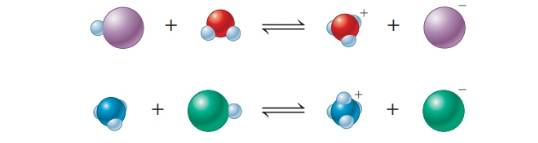Chapter 16, Problem 88AP### Introductory Chemistry: A Foundati...

9th Edition
Steven S. Zumdahl + 1 other
ISBN: 9781337399425

#### Solutions

Chapter
Section### Introductory Chemistry: A Foundati...

9th Edition
Steven S. Zumdahl + 1 other
ISBN: 9781337399425
Textbook Problem
5 views

# . The following are representations of acid-base reactions:a. Label each of the species in both equations as an acid or base, and explain. b. For those species that are acids, which labels apply: Arrhenius acid, Brønsted-Lowry acid? What about the bases?

Interpretation Introduction

(a)

Interpretation:

Each of the species in both equations should be labeled as an acid or base and should be explained.

Concept Introduction:

Acid is a substance that gives hydrogen ions in the aqueous solution and base gives hydroxide ions in the aqueous solution. This is based on Arrhenius concept of acids and bases.

An acid is also defined as a proton donor and base as a proton acceptor based on Bronsted-Lowry model.

Explanation

By looking at the charge of the products, we can determine which species is the acid and which is the base. Acids are proton donors...

Interpretation Introduction

(b)

Interpretation:

For those species that are acids, which are Arrhenius acids, and which are Bronsted-Lowry acids should be indicated. Same way the bases also should be specified.

Concept Introduction:

Acid is a substance that gives hydrogen ions in the aqueous solution and base gives hydroxide ions in the aqueous solution. This is based on Arrhenius concept of acids and bases.

An acid is also defined as a proton donor and base as a proton acceptor based on Bronsted-Lowry model.

### Still sussing out bartleby?

Check out a sample textbook solution.

See a sample solution

#### The Solution to Your Study Problems

Bartleby provides explanations to thousands of textbook problems written by our experts, many with advanced degrees!

Get Started